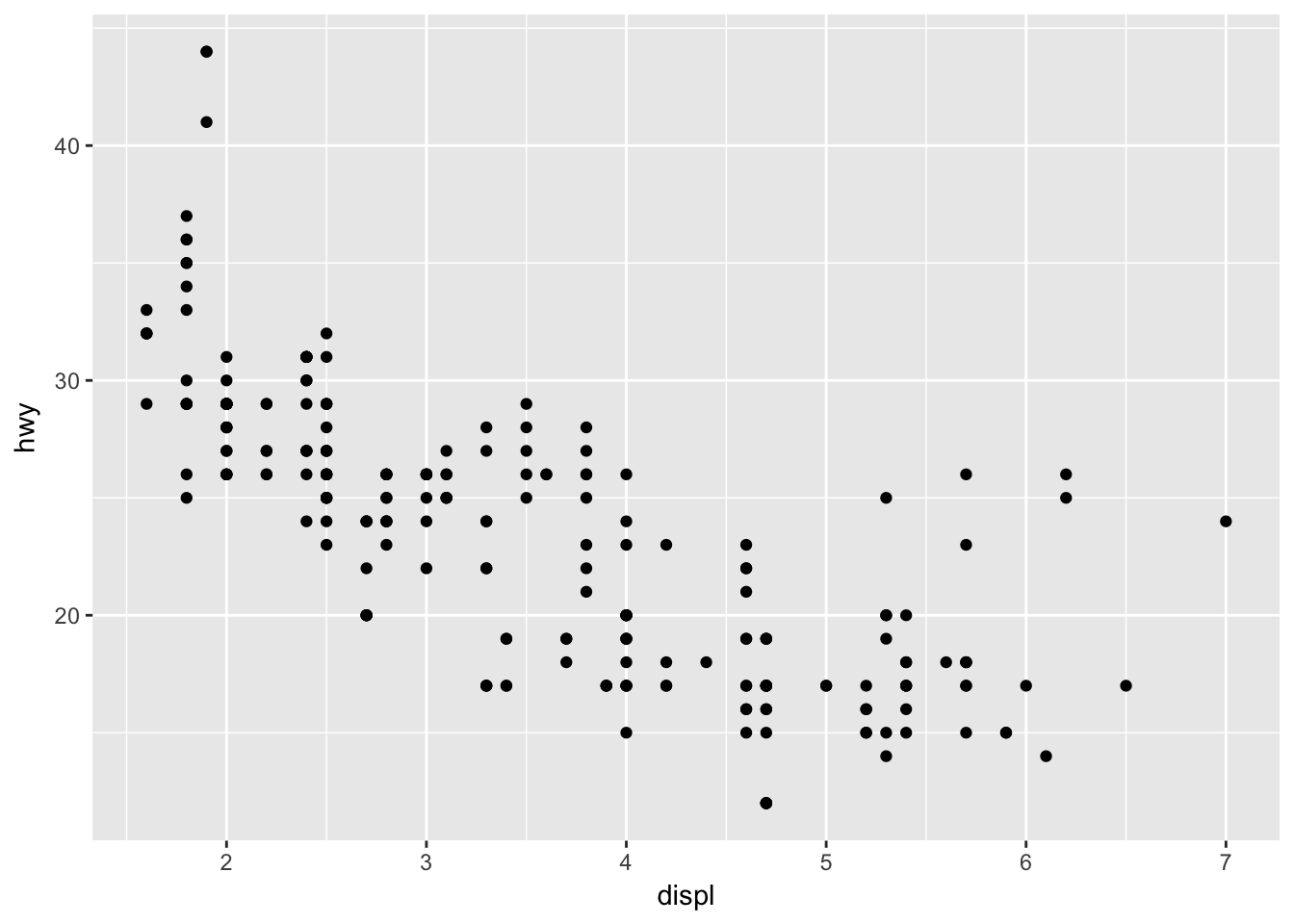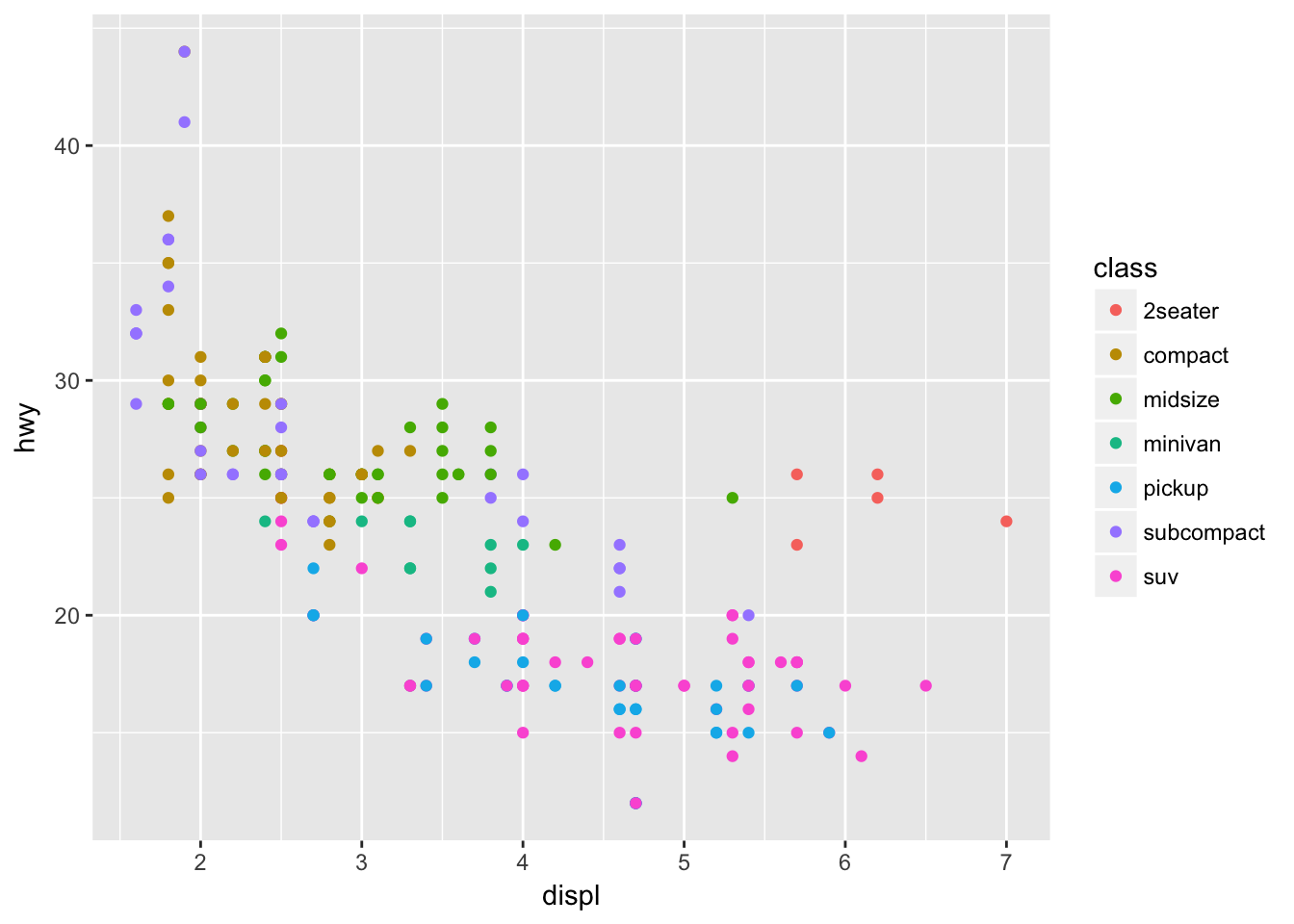``````library(ggplot2)
library(rgdal)
library(dplyr)
library(viridis)
library(broom)
library(dplyr)``````

## Overview

ggplot2 is a popular package for visualizing data in R.

ggplot2 is a system for declaratively creating graphics, based on The Grammar of Graphics. You provide the data, tell ggplot2 how to map variables to aesthetics, what graphical primitives to use, and it takes care of the details.

It’s been around for years and has pretty good documentation and tons of example code around the web (like on StackOverflow). This lesson will introduce you to the basic components of working with ggplot2.

## Lesson

### ggplot vs base vs lattice vs XYZ…

R provides many ways to get your data into a plot. Three common ones are,

• “base graphics” (`plot`, `hist`, etc`)
• lattice
• ggplot2

All of them work! I use base graphics for simple, quick and dirty plots. I use ggplot2 for most everything else.

ggplot2 excels at making complicated plots easy and easy plots simple enough.

### Geoms / Aesthetics

Every graphic you make in ggplot2 will have at least one aesthetic and at least one geom (layer). The aesthetic maps your data to your geometry (layer). Your geometry specifies the type of plot we’re making (point, bar, etc.).

``````ggplot(mpg, aes(displ, hwy)) +
geom_point()``````What makes ggplot really powerful is how quickly we can make this plot visualize more aspects of our data. Coloring each point by class (compact, van, pickup, etc.) is just a quick extra bit of code:

``````ggplot(mpg, aes(displ, hwy, color = class)) +
geom_point()``````Aside: How did I know to write `color = class`? `aes` will pass its arguments on to any geoms you use and we can find out what aesthetic mappings geom_point takes with `?geom_point` (see section “Aesthetics”)

Challenge: Find another aesthetic mapping `geom_point` can take and add add it to the plot.

What if we just wanted the color of the points to be blue? Maybe we’d do this:

``````ggplot(mpg, aes(displ, hwy, color = "blue")) +
geom_point()``````